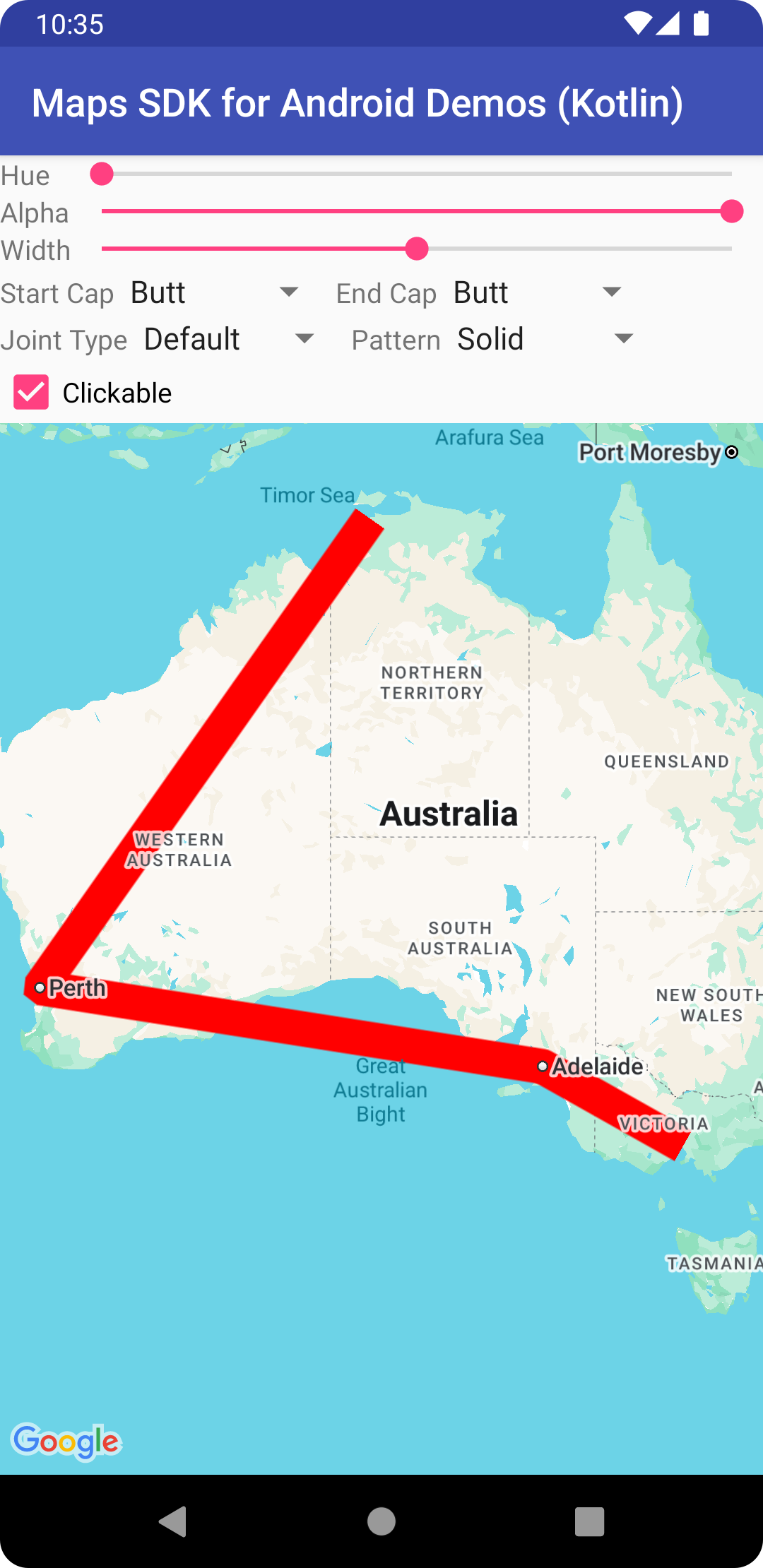# 形状Google Maps API for Android 为您提供了一些向地图添加形状的简单方法，以便针对您的应用对其进行自定义。

• `Polyline` 是一系列相连的线段，可组成您想要的任何形状，并可用于在地图上标记路径和路线。
• `Polygon` 是一种封闭形状，可用于在地图上标记区域。
• `Circle` 是在地图上绘制的投影图，表示一个圆形在地图表面的投影，具有地理准确性。

## 多段线

`Polyline` 类在地图上定义一组相连的线段。`Polyline` 对象包含一组 `LatLng` 位置，并可创建一系列线段，依照先后次序将这些位置连接起来。

### Java

```// Instantiates a new Polyline object and adds points to define a rectangle
PolylineOptions polylineOptions = new PolylineOptions()
.add(new LatLng(37.45, -122.0))  // North of the previous point, but at the same longitude
.add(new LatLng(37.45, -122.2))  // Same latitude, and 30km to the west
.add(new LatLng(37.35, -122.2))  // Same longitude, and 16km to the south
.add(new LatLng(37.35, -122.0)); // Closes the polyline.

// Get back the mutable Polyline
```

### Kotlin

```// Instantiates a new Polyline object and adds points to define a rectangle
val polylineOptions = PolylineOptions()
.add(LatLng(37.45, -122.0)) // North of the previous point, but at the same longitude
.add(LatLng(37.45, -122.2)) // Same latitude, and 30km to the west
.add(LatLng(37.35, -122.2)) // Same longitude, and 16km to the south
.add(LatLng(37.35, -122.0)) // Closes the polyline.

// Get back the mutable Polyline
```

## 多边形

`Polygon` 对象与 `Polyline` 对象类似，因为它们都包含一系列有序的坐标。不过，多边形并不是开放式的，它们用于定义环路内的区域，并且内部是实心的。

### Java

```// Instantiates a new Polygon object and adds points to define a rectangle
PolygonOptions polygonOptions = new PolygonOptions()
new LatLng(37.45, -122.0),
new LatLng(37.45, -122.2),
new LatLng(37.35, -122.2),
new LatLng(37.35, -122.0));

// Get back the mutable Polygon
```

### Kotlin

```// Instantiates a new Polygon object and adds points to define a rectangle
val rectOptions = PolygonOptions()
LatLng(37.35, -122.0),
LatLng(37.45, -122.0),
LatLng(37.45, -122.2),
LatLng(37.35, -122.2),
LatLng(37.35, -122.0)
)

// Get back the mutable Polygon
```

### Java

```Polygon polygon1 = map.addPolygon(new PolygonOptions()
new LatLng(0, 5),
new LatLng(3, 5),
new LatLng(0, 0))
.strokeColor(Color.RED)
.fillColor(Color.BLUE));

new LatLng(0, 5),
new LatLng(3, 5))
.strokeColor(Color.RED)
.fillColor(Color.BLUE));
```

### Kotlin

```val polygon1 = map.addPolygon(
PolygonOptions()
LatLng(0.0, 0.0),
LatLng(0.0, 5.0),
LatLng(3.0, 5.0),
LatLng(0.0, 0.0)
)
.strokeColor(Color.RED)
.fillColor(Color.BLUE)
)
PolygonOptions()
LatLng(0.0, 0.0),
LatLng(0.0, 5.0),
LatLng(3.0, 5.0)
)
.strokeColor(Color.RED)
.fillColor(Color.BLUE)
)
```

### Java

```List<LatLng> hole = Arrays.asList(new LatLng(1, 1),
new LatLng(1, 2),
new LatLng(2, 2),
new LatLng(2, 1),
new LatLng(1, 1));
new LatLng(0, 5),
new LatLng(3, 5),
new LatLng(3, 0),
new LatLng(0, 0))
.fillColor(Color.BLUE));
```

### Kotlin

```val hole = listOf(
LatLng(1.0, 1.0),
LatLng(1.0, 2.0),
LatLng(2.0, 2.0),
LatLng(2.0, 1.0),
LatLng(1.0, 1.0)
)
PolygonOptions()
LatLng(0.0, 0.0),
LatLng(0.0, 5.0),
LatLng(3.0, 5.0),
LatLng(3.0, 0.0),
LatLng(0.0, 0.0)
)
.fillColor(Color.BLUE)
)
```

## 圆形

• `center`，以 `LatLng` 形式表示。
• `radius`（以米为单位）。

### Java

```// Instantiates a new CircleOptions object and defines the center and radius
CircleOptions circleOptions = new CircleOptions()
.center(new LatLng(37.4, -122.1))

// Get back the mutable Circle
```

### Kotlin

```// Instantiates a new CircleOptions object and defines the center and radius
val circleOptions = CircleOptions()
.center(LatLng(37.4, -122.1))

// Get back the mutable Circle
```

### Java

```Circle circle = map.addCircle(new CircleOptions()
.center(new LatLng(37.4, -122.1))
.strokeWidth(10)
.strokeColor(Color.GREEN)
.fillColor(Color.argb(128, 255, 0, 0))
.clickable(true));

@Override
public void onCircleClick(Circle circle) {
// Flip the r, g and b components of the circle's stroke color.
int strokeColor = circle.getStrokeColor() ^ 0x00ffffff;
circle.setStrokeColor(strokeColor);
}
});
```

### Kotlin

```val circle = map.addCircle(
CircleOptions()
.center(LatLng(37.4, -122.1))
.strokeWidth(10f)
.strokeColor(Color.GREEN)
.fillColor(Color.argb(128, 255, 0, 0))
.clickable(true)
)
map.setOnCircleClickListener {
// Flip the r, g and b components of the circle's stroke color.
val strokeColor = it.strokeColor xor 0x00ffffff
it.strokeColor = strokeColor
}
```

## 自定义外观

### Java

```Polyline polyline = map.addPolyline(new PolylineOptions()
.add(new LatLng(-37.81319, 144.96298), new LatLng(-31.95285, 115.85734))
.width(25)
.color(Color.BLUE)
.geodesic(true));
```

### Kotlin

```val polyline = map.addPolyline(
PolylineOptions()
.width(25f)
.color(Color.BLUE)
.geodesic(true)
)
```

### Java

```List<PatternItem> pattern = Arrays.asList(
new Dot(), new Gap(20), new Dash(30), new Gap(20));
polyline.setPattern(pattern);
```

### Kotlin

```val pattern = listOf(
Dot(), Gap(20F), Dash(30F), Gap(20F)
)
polyline.pattern = pattern
```

### Java

```polyline.setJointType(JointType.ROUND);
```

### Kotlin

```polyline.jointType = JointType.ROUND
```

### Java

```polyline.setStartCap(new RoundCap());
```

### Kotlin

```polyline.startCap = RoundCap()
```

### Java

```polyline.setEndCap(
new CustomCap(BitmapDescriptorFactory.fromResource(R.drawable.arrow), 16));
```

### Kotlin

```polyline.endCap = CustomCap(BitmapDescriptorFactory.fromResource(R.drawable.arrow), 16F)
```

### Z-index

Z-index 指定该形状相对于地图上其他叠加层（其他形状、地面叠加层和图块叠加层）的叠放顺序。Z-index 较高的叠加层在 Z-index 较低的叠加层之上绘制。Z-index 相同的两个叠加层按任意顺序绘制。

## 将数据与形状关联

### Java

```Polyline polyline = map.addPolyline((new PolylineOptions())
.clickable(true)
new LatLng(-34.747, 145.592),
new LatLng(-34.364, 147.891),
new LatLng(-33.501, 150.217),
new LatLng(-32.306, 149.248),
new LatLng(-32.491, 147.309)));

polyline.setTag("A");
```

### Kotlin

```val polyline = map.addPolyline(
PolylineOptions()
.clickable(true)
LatLng(-35.016, 143.321),
LatLng(-34.747, 145.592),
LatLng(-34.364, 147.891),
LatLng(-33.501, 150.217),
LatLng(-32.306, 149.248),
LatLng(-32.491, 147.309)
)
)
polyline.tag = "A"
```

• 您的应用可能适合不同类型的形状，并且您希望在用户点击形状时通过不同方式处理它们。
• 您可能会与具有唯一记录标识符的系统进行互动，其中形状表示该系统中的特定记录。
• 形状数据可能会指明用于确定形状 Z-index 的优先级。
[{ "type": "thumb-down", "id": "missingTheInformationINeed", "label":"没有我需要的信息" },{ "type": "thumb-down", "id": "tooComplicatedTooManySteps", "label":"太复杂/步骤太多" },{ "type": "thumb-down", "id": "outOfDate", "label":"内容需要更新" },{ "type": "thumb-down", "id": "translationIssue", "label":"翻译问题" },{ "type": "thumb-down", "id": "samplesCodeIssue", "label":"Samples / code issue" },{ "type": "thumb-down", "id": "otherDown", "label":"其他" }]
[{ "type": "thumb-up", "id": "easyToUnderstand", "label":"易于理解" },{ "type": "thumb-up", "id": "solvedMyProblem", "label":"解决了我的问题" },{ "type": "thumb-up", "id": "otherUp", "label":"其他" }]